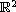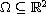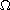Electron. J. Diff. Equ., Vol. 2011 (2011), No. 100, pp. 1-54.

### Stability and approximations of eigenvalues and eigenfunctions of the Neumann Laplacian, part 3 Michael M. H. Pang

Abstract:
This article is a sequel to two earlier articles (one of them written jointly with R. Banuelos) on stability results for the Neumann eigenvalues and eigenfunctions of domains inwith a snowflake type fractal boundary. In particular we want our results to be applicable to the Koch snowflake domain. In the two earlier papers we assumed that a domainwhich has a snowflake type boundary is approximated by a family of subdomains and that the Neumann heat kernel ofand those of its approximating subdomains satisfy a uniform bound for all sufficiently small t>0. The purpose of this paper is to extend the results in the two earlier papers to the situations where the approximating domains are not necessarily subdomains of. We then apply our results to the Koch snowflake domain when it is approximated from outside by a decreasing sequence of polygons.

Submitted November 5, 2010. Published August 7, 2011.
Math Subject Classifications: 35P05, 35P15.
Key Words: Stability; approximations; Neumann eigenvalues and eigenfunctions.

Show me the PDF file (525 KB), TEX file, and other files for this article.Michael M. H. Pang Department of Mathematics, University of Missouri-Columbia Columbia, MO 65211, USA email: pangm@missouri.edu